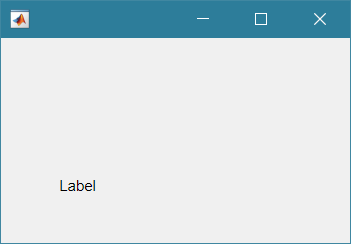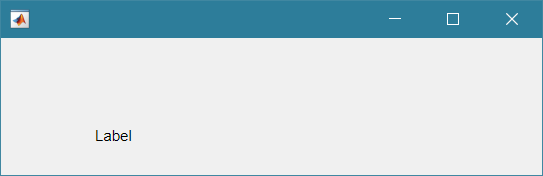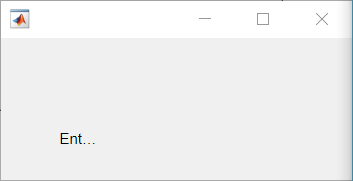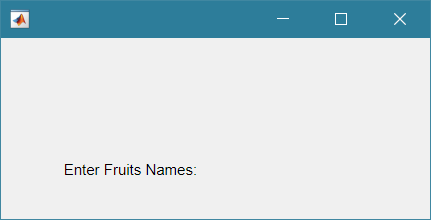# Label component in MATLAB GUI

• Last Updated : 22 Sep, 2021

Matlab is a numeric computing environment that comes with its own programming language. It specializes in technical computing in areas like Science, Engineering, and many others. Matlab also provides the ability to create GUI applications by simply using its functions for various GUI components.

In this article, we will learn about the Label component, how to create one, and its important properties.

## Label Component

A label is a UI component that holds the static text to label different parts of an application. It is useful in GUI applications as it describes the different parts of the UI. Matlab provides a function called uilabel to create a label. There are three syntaxes that can be used:

• labelObject = uilabel
• labelObject = uilabel(parent)
• labelObject = uilabel(parent, Name, Value)

## Properties of Uilabel component

To control the appearances and behaviors of the component, Matlab provides many properties. Some important properties are as follows:

• Text: The text property is used to control the content of the label. The default value is ‘Label’.
• Interpreter: This property allows us to interpret the text of the label using a different interpreter. Like we can use latex code for writing equations or HTML for formatting as text and set the interpreter to be ‘latex’ or ‘HTML’. Default is ‘none.
• HorizontalAlignment: Controls the horizontal alignment of the text within the label component. Default is ‘left’.
• VerticalAlignment: Controls the vertical alignment within the component. Default is ‘center’.
• Wordwrap: Wraps the text to fit the component’s width. Default is ‘off’.
• FontName: changes the font for the text.
• FontSize: controls the font size.
• font-weight: controls the weight/boldness of the text.
• FontAngle: controls the font angle.
• font color: the color of the font.
• BackgroundColor: change the background colour of the label.
• Visible: This property controls the visibility of the component. Default is ‘on’.
• Enable: Enable or Disabled appearance. Default is ‘on’.
• TootTip: A text to guide the purpose of the component. Default is ”.

## Uilabel()

The first syntax does not need any parameters. It is created with “Label” as the default text. Matlab creates a figure window and stores the label into the window for us.

Example:

## Matlab

 `% MATLAB code for Uilabel() demonstration.``% create a uilabel using only the function``label = uilabel;`

Output:## uilabel(parent)

Matlab also provides the option to pass a custom window as the parent of the component.

Example:

## Matlab

 `% MATLAB code for Uilabel(parent) demonstration.``% create a figure``fig = uifigure;`` ` `% create a label and pass the figure as parent``label = uilabel(fig);`

Output:## uilabel(parent, Name, Value)

Matlab also provides us with the option to pass the value to the component using name-value pairs.

Example:

## Matlab

 `%  MATLAB code for uilabel(parent, Name, Value) function``% create a figure``fig = uifigure;`` ` `% create a label and pass the figure as parent``label = uilabel(fig, ``'Text'``, ``'Enter Fruits Names:'``);`

Output:We can see that the size of the component is small and hence the text is clipped. We can fix the issue by modifying the size of the label component.

Example:

## Matlab

 `% create a figure``fig = uifigure;`` ` `% create a label and pass the figure as parent``label = uilabel(fig, ``'Text'``, ``'Enter Fruits Names:'``);`` ` `% changing the size of the  ``label.Position(3:4) = [120, 22];`

Output:The position property is a 4 valued list where the first 2 values are the positions and the last 2 values are the sizes of the component. In the code above we have changed the value of the last two indices (3:4).

My Personal Notes arrow_drop_up